## Method

Dense 6D Visual Odometry [st] [D6DVO]

Submitted on 20 Nov. 2012 00:32 by
Maxime Meilland (I3S-CNRS)

 Running time: 0.03 s Environment: 1 core @ 2.5 Ghz (C/C++)

 Method Description: Dense Stereo Visual Odometry. The method is based on a direct registration of the entire images. Dense depth-maps are obtained by stereo matching on CPU (block matching), while dense registration is performed simultaneously on GPU (OpenCL). Huber influence function is used for robust outliers rejection. Contrary to the original approach [Comport07] only the left camera is used for localisation, and the image warping is directly performed in 3D. Parameters: \blockMatchingSize 9\maxDisparity 128\imagePyramidSize 4 Latex Bibtex: @INPROCEEDINGS{Comport07,title={Accurate Quadrifocal Tracking for Robust 3D Visual Odometry},author={Comport, Andrew Ian and Malis, E. and Rives, P.},booktitle={ICRA},year={2007},}@INPROCEEDINGS{Meilland11a,author={Meilland, M. and Comport, A. I. and Rives, P.},booktitle={ICRA},title={Dense visual mapping of large scale environments for real-time localisation},year={2011},}

## Detailed Results

From all test sequences (sequences 11-21), our benchmark computes translational and rotational errors for all possible subsequences of length (5,10,50,100,150,...,400) meters. Our evaluation ranks methods according to the average of those values, where errors are measured in percent (for translation) and in degrees per meter (for rotation). Details for different trajectory lengths and driving speeds can be found in the plots underneath. Furthermore, the first 5 test trajectories and error plots are shown below.

## Test Set Average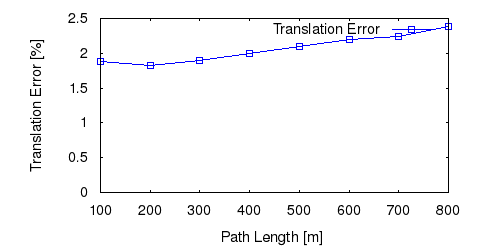This figure as: png eps pdf txt gnuplotThis figure as: png eps pdf txt gnuplot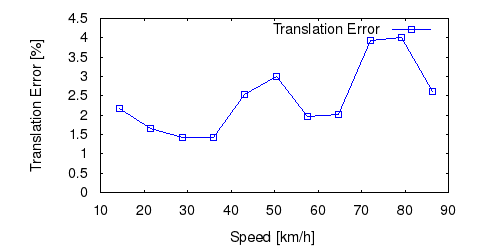This figure as: png eps pdf txt gnuplot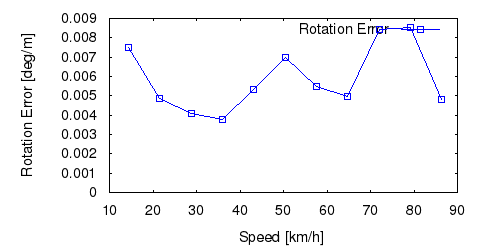This figure as: png eps pdf txt gnuplot

## Sequence 11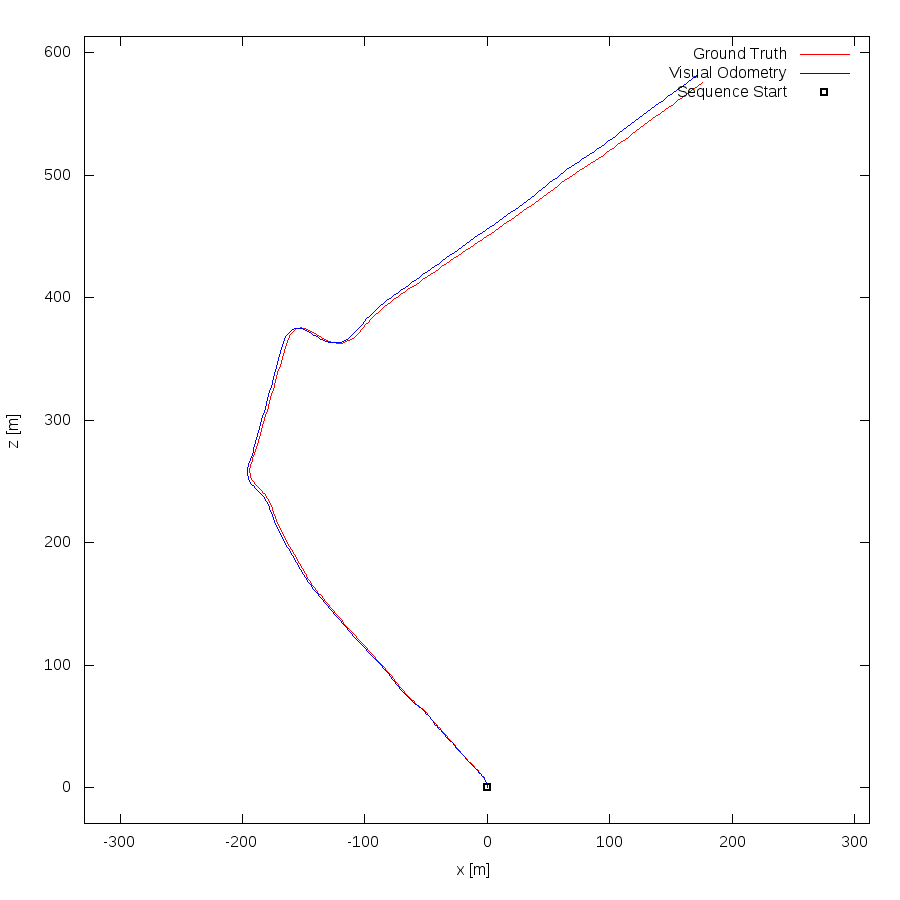This figure as: png eps pdf txt gnuplot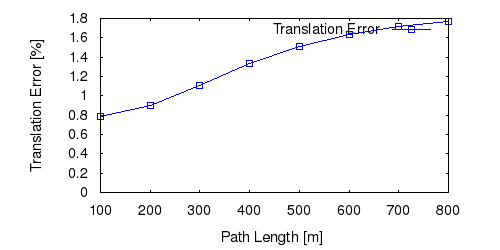This figure as: png eps pdf txt gnuplot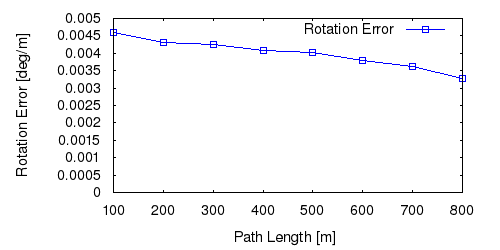This figure as: png eps pdf txt gnuplot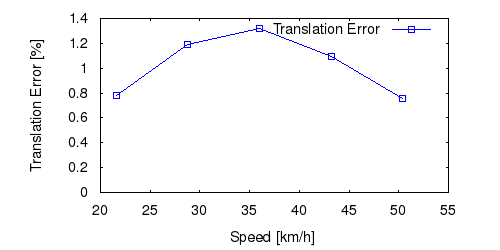This figure as: png eps pdf txt gnuplot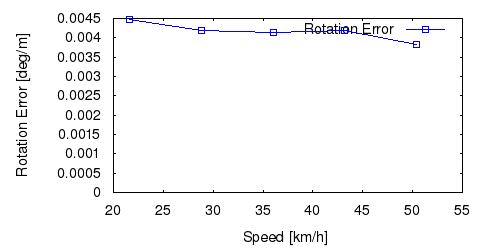This figure as: png eps pdf txt gnuplot

## Sequence 12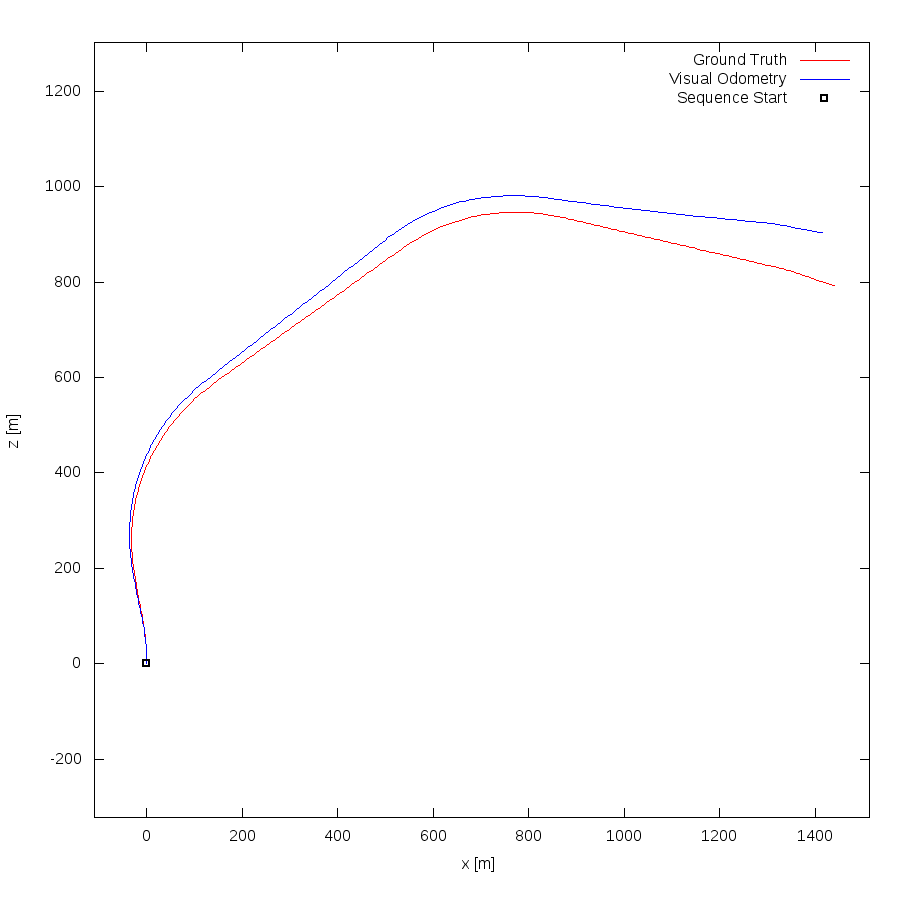This figure as: png eps pdf txt gnuplot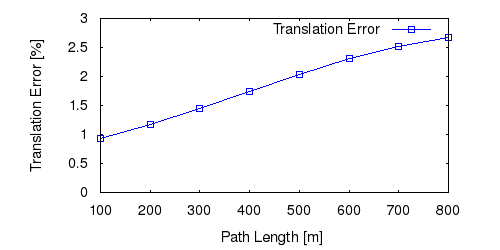This figure as: png eps pdf txt gnuplot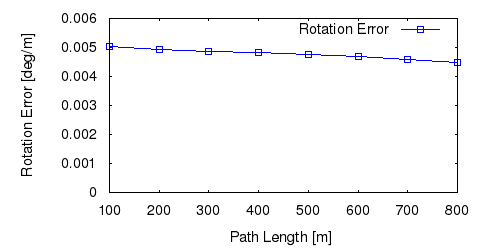This figure as: png eps pdf txt gnuplotThis figure as: png eps pdf txt gnuplotThis figure as: png eps pdf txt gnuplot

## Sequence 13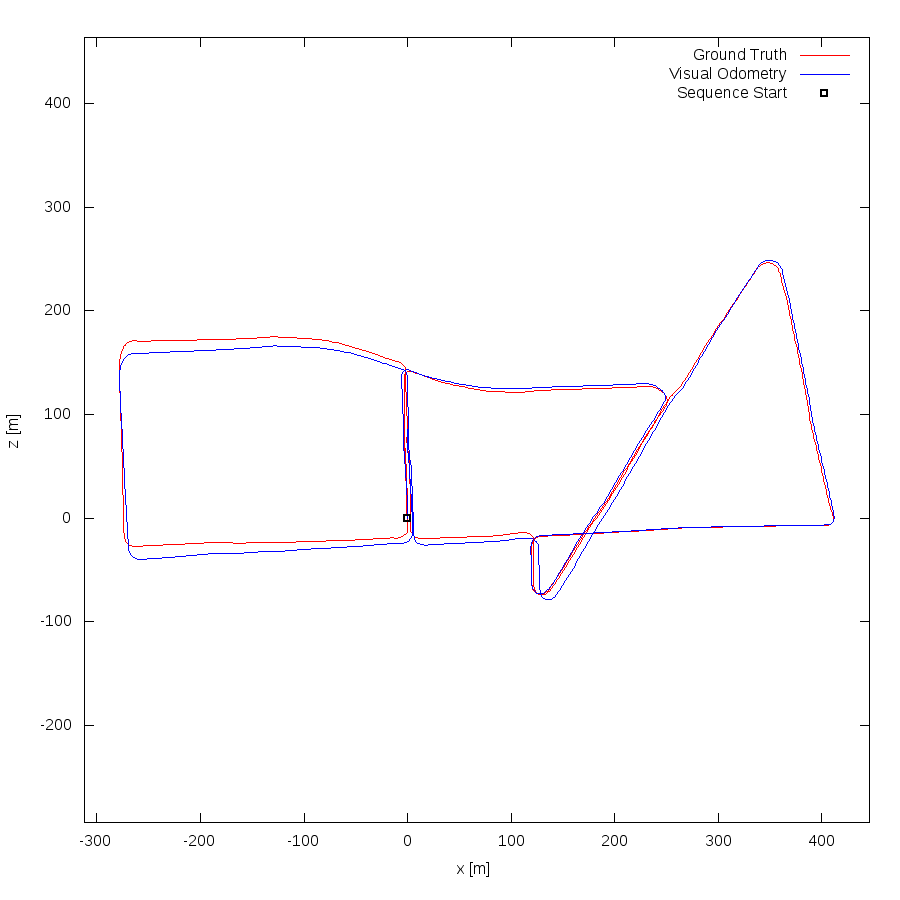This figure as: png eps pdf txt gnuplot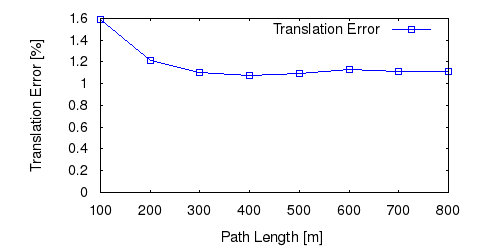This figure as: png eps pdf txt gnuplot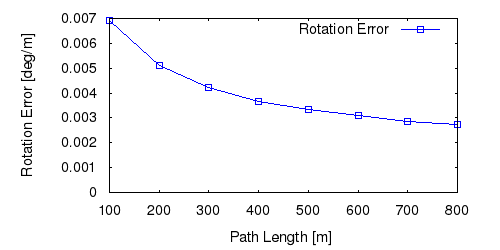This figure as: png eps pdf txt gnuplotThis figure as: png eps pdf txt gnuplot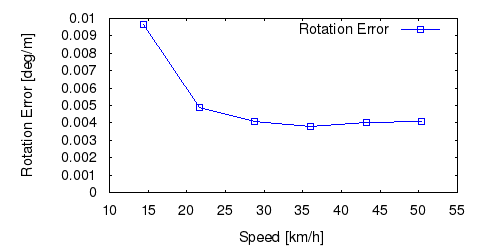This figure as: png eps pdf txt gnuplot

## Sequence 14This figure as: png eps pdf txt gnuplot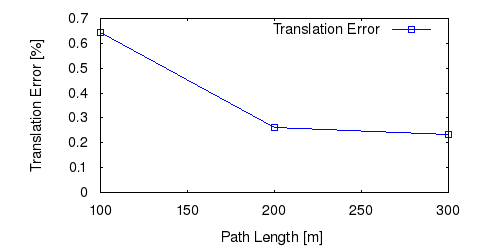This figure as: png eps pdf txt gnuplot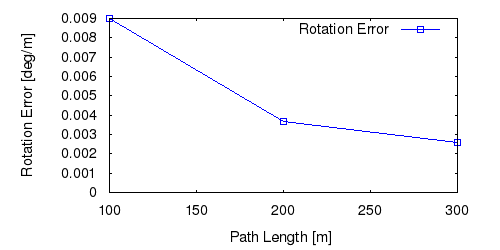This figure as: png eps pdf txt gnuplotThis figure as: png eps pdf txt gnuplot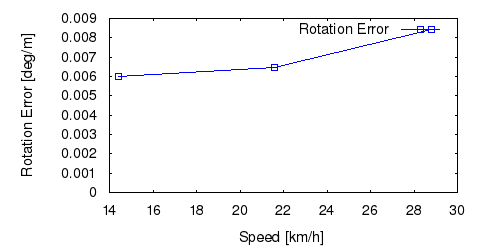This figure as: png eps pdf txt gnuplot

## Sequence 15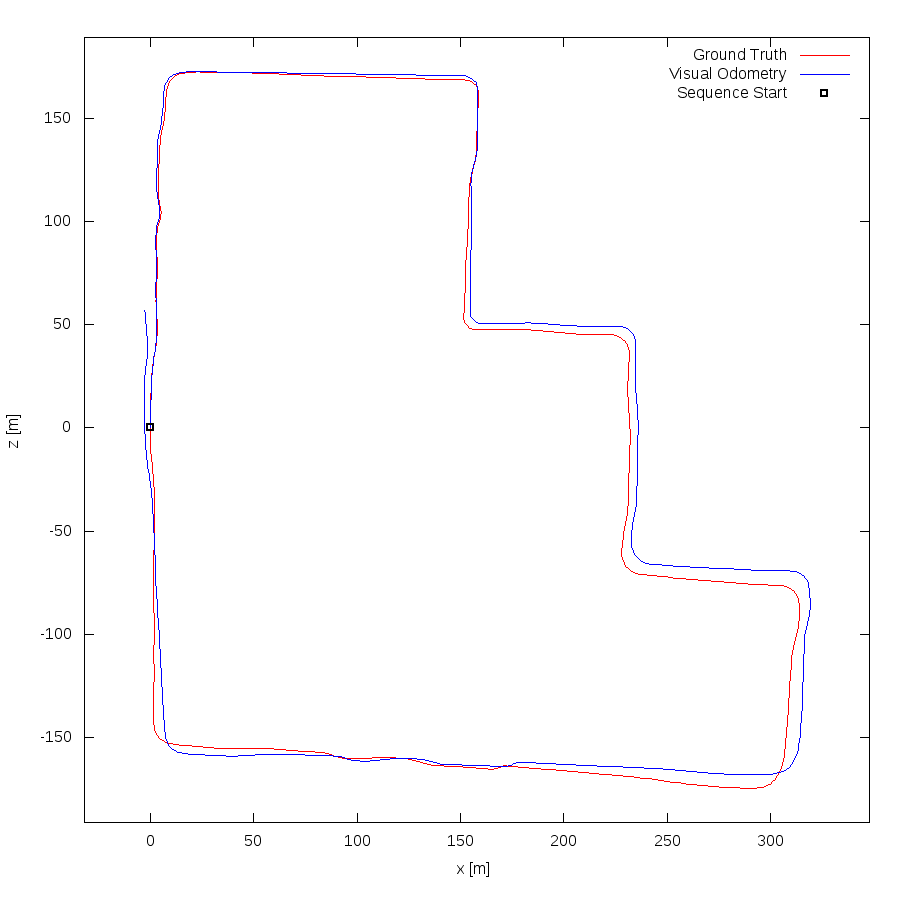This figure as: png eps pdf txt gnuplot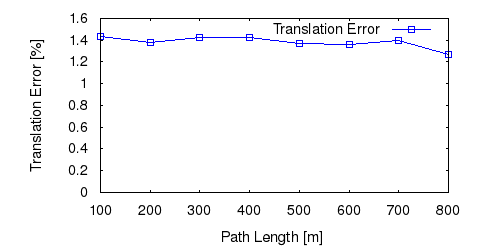This figure as: png eps pdf txt gnuplotThis figure as: png eps pdf txt gnuplot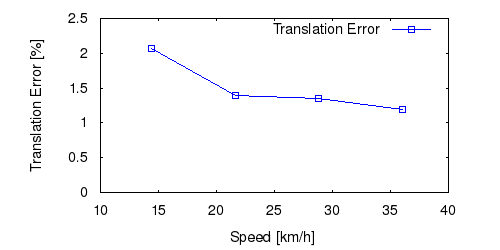This figure as: png eps pdf txt gnuplotThis figure as: png eps pdf txt gnuplot